# Using Excel and R to simulate coin flipping by @CambridgeMaths

Previously, we discussed how macros in Excel can be used to create simulations for repeated dice rolls. While the basic macro coding skills covered in those blogs are useful for producing slick, pre-created examples, they do require a significant investment of time and effort to produce. This blog explores an alternative approach to producing simulated data in Excel and then using R to analyse it. The data to be simulated is the process of flipping five coins and counting the number of heads.

The first step is to mathematise the act of flipping a coin: the easiest way to do this is to assign a score of 0 for a tail and 1 for a head. In Excel, type into a cell =RANDBETWEEN(0,1) and it will generate randomly either a 1 or a 0. In essence, that is the simulation built. In the case of five concurrent coin flips, simply copy the formula into five adjacent cells and to get a count of the number of heads, use the sum command =SUM(A2:E2) to add the coin flips. To generate a set of results simply highlight all 6 cells, then click and drag the formulae down by using the small “plus” sign in the bottom right corner of the highlight box.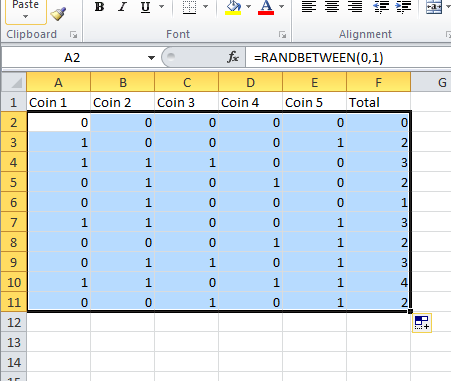It’s not necessary to name the columns, but it does make the commands in R a little simpler if you do.

The next step is to save the data in a format that can be read by R. Choose save as CSV and save the data to your R working directory . In the example, we will assume the file is saved as coins.csv

Now open R and get ready to import the data. Enter the following command: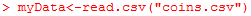This reads the data from the csv file and stores it in R in an object called myData; typing myData and hitting enter will show the dataset on screen.

For the graph, you are only interested in the total column, which can be accessed by typing myData\$Total. This is the reason we named the columns in the original spreadsheet, as by default, R will assign columns names such as X1.1 if it is forced to make something up.

It can be useful to assign the column to a more simply named data object, and it would also be helpful to have the data in the form of a table, rather than a list of raw data. We can do both of these in a single command by typing in: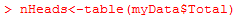The table() command creates a frequency table from the named object inside the parenthesis and nHeads is the name of this new object.

Typing nHeads will show the table.

To draw a graph simply type plot(nHeads) or barplot(nHeads) depending on whether you prefer a bar chart or a line graph. To change the axis labels and title, a few extra parameters main, xlab and ylab can be added as follows: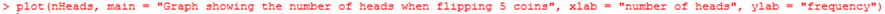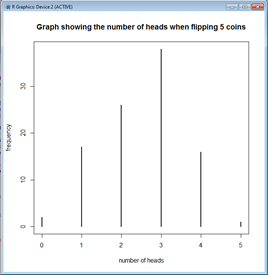And that’s it! You can set everything up in advance of a lesson by typing the commands into a notepad app, and then copy and pasting everything to R during the lesson.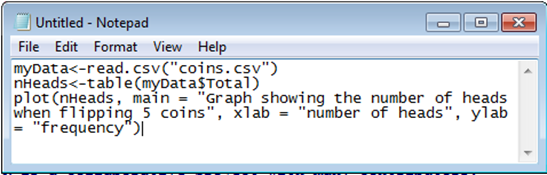To update the data, hit F9 in Excel to generate new random numbers, save the file, and then run through the R commands again to show how the precise data changes but the aggregate shape is fairly consistent.

Try varying the number of coins, or the number of simulated trials and see what happens. For a small number of trials, in order to see the individual data, using the graph function stripchart() may be preferable to using plot()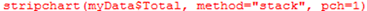In this case, the raw data from the total column is used, method=”stack” formats the dot plot into vertical bars and pch=1 draws circles for each data point which look much nicer than the default squares.

In this example, the data used came from a simulation, but any similarly structured dataset recorded in Excel will work equally well.